# Lecture Notes in Deep Learning: Unsupervised Learning – Part 1

## Motivation & Restricted Boltzmann Machines

These are the lecture notes for FAU’s YouTube Lecture “Deep Learning“. This is a full transcript of the lecture video & matching slides. We hope, you enjoy this as much as the videos. Of course, this transcript was created with deep learning techniques largely automatically and only minor manual modifications were performed. Try it yourself! If you spot mistakes, please let us know!

Welcome back to deep learning! So today, we want to talk about unsupervised methods and in particular, we will focus on autoencoders and GANs in the next couple of videos. We will start today with the basics, the motivation, and look into one of the rather historical methods – the restricted Boltzmann machines. We still mention them here, because they are kind of important in terms of the developments towards unsupervised learning.

So, let’s see what I have here for you. So, the main topic as I said is unsupervised learning. Of course, we start with our motivation. So, you see that the data sets we’ve seen so far, they are huge they had up to millions of different training observations, many objects, and in particular few modalities. Most of the things we’ve looked at where essentially camera images. There may have been different cameras that have been used but typically only one or two modalities that were in one single dataset. However, this is not generally the case. For example, in medical imaging, you have typically very small data sets, maybe 30 to 100 patients. You have only one complex object that is the human body and many different modalities from MR, X-ray, to ultrasound. All of them have a very different appearance which means that they also have different requirements in terms of their processing. So why is this the case? Well in Germany, we actually have 65 CT scans per thousand inhabitants. This means that in 2014 alone, we had five million CT scans in Germany. So, there should be plenty of data. Why can’t we use all of this data? Well, these data are, of course, sensitive and they contain patient health information. So, for example, if you have a scan that contains the head in a CT scan, then you can render the surface of the face and you can even use an automatic system to determine the identity of this person. There are also non-obvious cues. So, for example, if you have the surface of the brain, the surface is actually characteristic for a certain person. You can identify persons by the shape of their brain to an accuracy of up to 99 percent. So, you see that this is indeed highly sensitive data. If you share whole volumes, people may be able to identify the person, although, you may argue that it’s difficult to identify a person from a single slice image. So, there are some trends to make data like this available. But still, you have the problem even if you have the data, you need labels. So, you need experts who look at the data and tell you what kind of disease is present, which anatomical structure is where, and so on. This is also very expensive to obtain.

So, it would be great if we had methods that could work with very few annotations or even no annotations. I have some examples here that go in this direction. One trend is weakly supervised learning. So, here you have a label for related tasks. The example that we show here is the localization from the class label. So let’s say, you have images and you have classes like brushing teeth or cutting trees. Then, you can use these plus the associated gradient information, like using visualization mechanisms, and you can localize the class in that particular image. This is a way how you can get a very cheap label, for example, for bounding boxes. There are also semi-supervised techniques where you have very little labeled data and you try to apply it to a larger data set. So, the typical approach here would be things like bootstrapping. You create a weak classifier from a small labeled data set. Then, you apply it to a large data set and you try to estimate which of the data points in that large data set have been classified reliably. Next, you take the reliable ones into a new training set and with the new training set, you then start over again trying to build a new system. Finally, you iterate until you have a better system.

Of course, there are also unsupervised techniques where you don’t need any labeled data. This will be the main topic of the next couple of videos. So let’s have a look at label-free learning. One typical application here is dimensionality reduction. Here, you have an example where data is on a high dimensional space. We have a 3-D space. Actually, we’re just showing you one slice through this 3-D space. You see that the data is rolled up and we identify similar points by similar color in this image. You can see this 3-D manifold that is often called the Swiss roll. Now, the Swiss roll actually doesn’t need a 3-D representation. So, what you would like to do is automatically unroll it. You see that here on the right-hand side, the dimensionality is reduced. So, you only have two dimensions here. This has been done automatically using a manifold learning technique or dimensionality reduction technique that is nonlinear. With these nonlinear methods, you can break down data sets into lower dimensionality. Now, this is useful because the smaller dimensionality is supposed to carry all the information that you need and you can now use this as a kind of representation.

What we’ll also see in the next couple of videos is that you can use this for example as network initialization. You already see the first auto-encoder structure here. You train such a network with a bottleneck where you have a low dimensional representation. Later, you take this low-dimensional representation and repurpose it. This means that you essentially remove the right-hand part of the network and replace it with a different one. Here, we use it for classification, and again our example is classifying cats and dogs. So, you can already see that if we are able to do such a dimensionality reduction, preserve the original information in a low dimensional space, then we potentially have fewer weights that we have to work with to approach a classification task. By the way, this is very similar to what we have already discussed when talking about transfer learning techniques.

You can also use this for clustering and you have already seen that. We have been using this technique in the chapter on visualization where we had this very nice dimensionality reduction and we zoomed in and looked over the different places here.

You’ve seen that if you have a good learning method that will extract a good representation, then you can also use it to identify similar images in such a low dimensional space. Well, this can also be used for generative models. So here, the task is to generate realistic images. You can tackle for example missing data problems with this. This then leads into semi-supervised learning where you can also use this, for example, for augmentation. You can also use it for image-to-image translation which is also a very cool application. We will later see the so-called cycle GAN where you can really do a domain translation. You can also use this to simulate possible futures in reinforcement learning. So, we would have all kinds of interesting domains where we could apply these unsupervised techniques as well. So, here are some examples of data generation. You train with the left-hand side and then you generate on the right-hand side those images. This would be an appealing thing to do. You could generate images that look like real observations.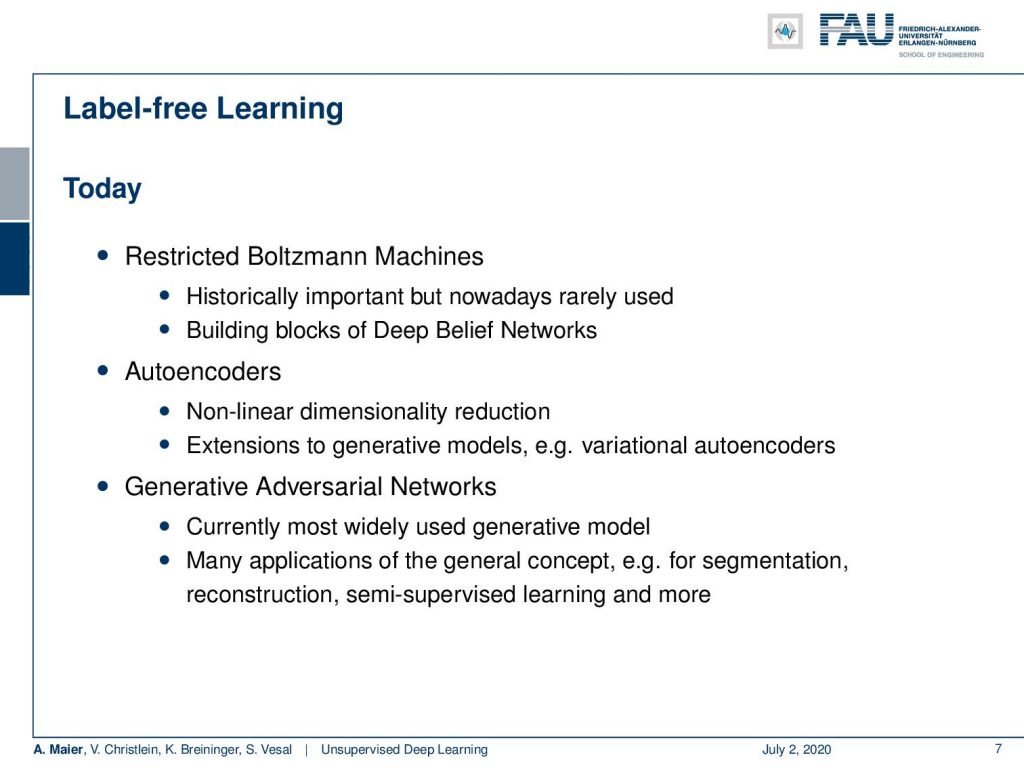Overview on the next couple of topics in our class. Image under CC BY 4.0 from the Deep Learning Lecture.

So today, we will talk about the restricted Boltzmann machines. As already indicated, they are historically important. But, honestly, nowadays they are not so commonly used anymore. They have been part of the big breakthroughs that we’ve seen earlier. For example, in Google dream. So, I think you should know about these techniques.

Later, we’ll talk about autoencoders which are essentially an emerging technology and kind of similar to the restricted Boltzmann machines. You can use them in a feed-forward network context. You can use them for nonlinear dimensionality reduction and even extend this to generative models like the variational auto-encoders which is also a pretty cool trick. Lastly, we will talk about general adversarial networks which are currently probably the most widely used generative models. There are many applications of this very general concept. You can use it in image segmentation, reconstruction, semi-supervised learning, and many more.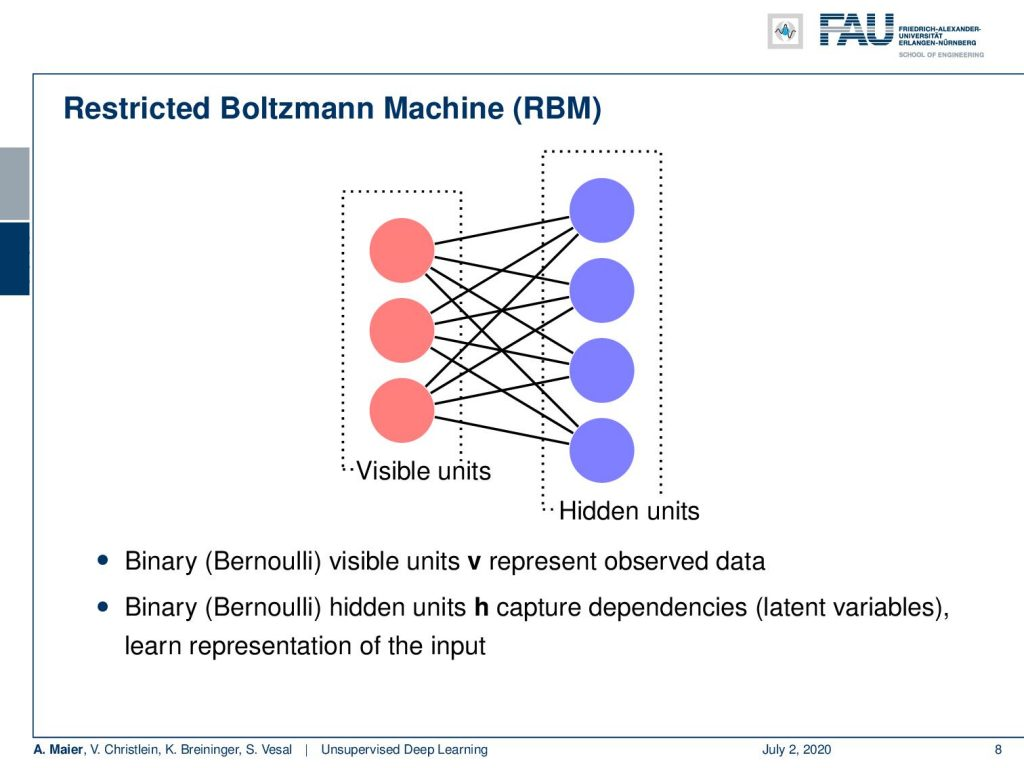Overview on the restricted Boltzmann machine. Image under CC BY 4.0 from the Deep Learning Lecture.

But let’s first look at the historical perspective. Probably these historical things like restricted Boltzmann machines are not so important if you encounter an exam with me at some point. Still, I think you should know about this technique. Now, the idea is a very simple one. So, you start with two sets of nodes. One of them consists of visible units and the other one of the hidden units. They’re connected. So, you have the visible units v and they represent the observed data. Then, you have the hidden units that capture the dependencies. So they’re latent variables and they’re supposed to be binary. So they’re supposed to be zeros and ones. Now, what can we do with this bipartite graph?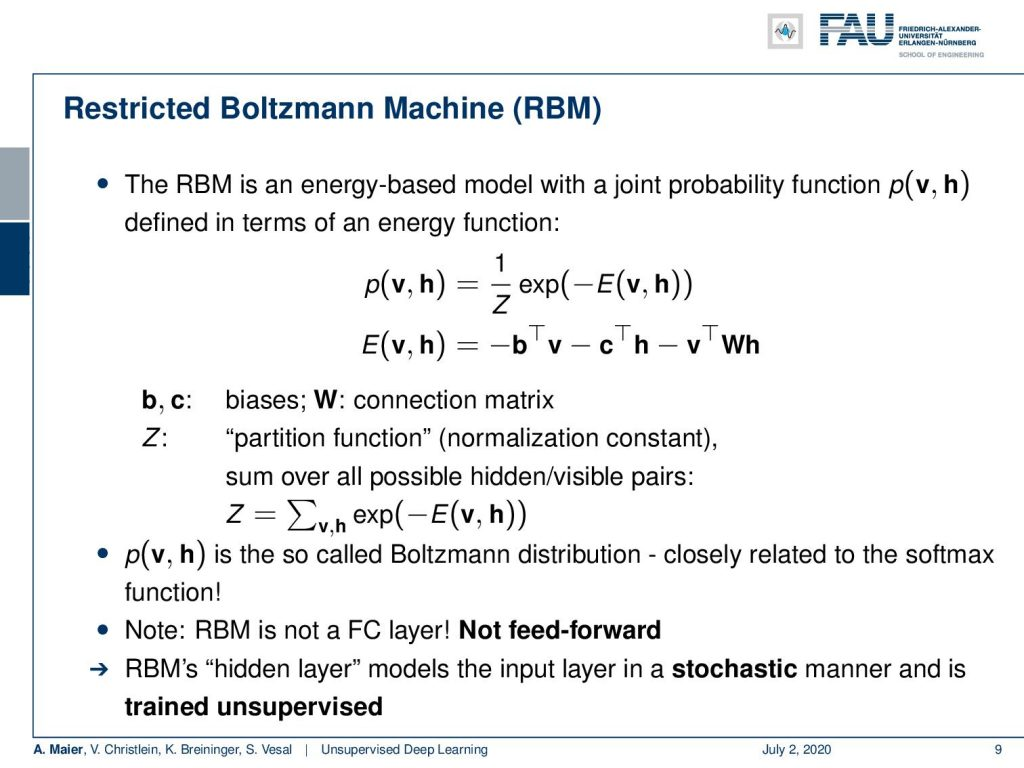RBMs seek to maximise the Boltzmann distribution. Image under CC BY 4.0 from the Deep Learning Lecture.

Well, you can see that the restricted Boltzmann machine is based on an energy model with a joint probability function that is p(v, h). It’s defined in terms of an energy function and this energy function is used inside the probability. So, you have 1/Z which is a kind of normalization constant. Then, e to the power of -E(v, h). The energy function that we’re defining here E(v, h) is essentially an inner product of the bias with v another bias and inner product with h and then a weighted inner product of v and h that is weighted with the matrix W. So, you can see that the unknowns here essentially are b, c, and the matrix W. So, this probability density function is called the Boltzmann distribution. It’s closely related to the softmax function. Remember that this is not simply a fully connected layer, because it’s not feed-forward. So, you feed into the restricted Boltzmann machines, you determine the h, and from the h you can then produce v again. So, the hidden layer model the input layer in a stochastic manner and is trained unsupervised.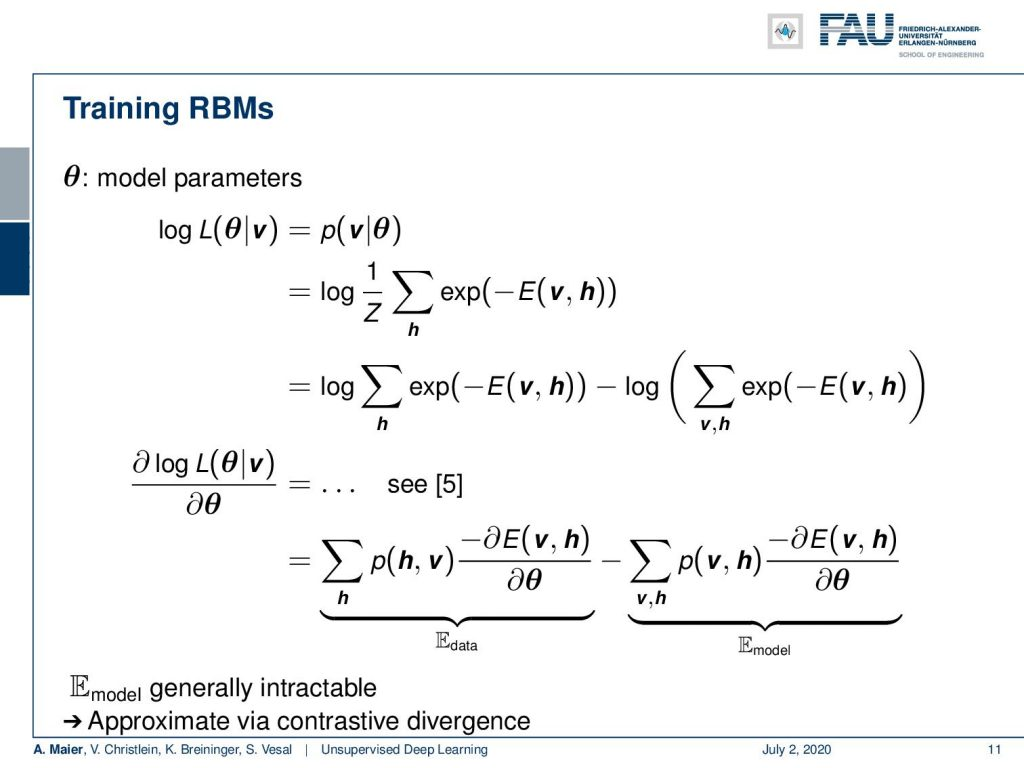We derive the training procedure using the log-likelihood of the Boltzmann distribution. Image under CC BY 4.0 from the Deep Learning Lecture.

So let’s look into some details here. The visible and hidden units form this bipartite graph as I already mentioned. You could argue that our RBMs are Markov random fields with hidden variables. Then, we want to find W such that our probability is high for low energy states and vice-versa. The learning is based on gradient descent on the negative log-likelihood. So, we start with the log-likelihood and you can see there’s a small mistake on this slide. We are missing a log in the p(v, h. We already fixed that in the next line where we have the logarithm of 1/Z and the sum of the exponential functions. Now, we can use the definition of Z and expand it. This allows us then to write this multiplication as a second logarithmic term. Because it’s 1/Z it’s -log the definition of Z. This is the sum over v and h over the exponential function of -E(v, h). Now, if we look at the gradient, you can see that the full derivation is given in . What you essentially get are two sums here. One is the sum over the p(h, v) times the negative partial differential of the energy function concerning the parameters minus the sum over v and h of the p(v, h) times the negative partial derivative of the energy function with respect to the parameters. Again, you can interpret those two terms as the expected value of the data and the expected value of the model. Generally, the expected value of the model is intractable, but you can approximate this with the so-called contrastive divergence.

Now, contrastive divergence works the following way: You take any training example as v. Then, you set the binary states of the hidden units by computing the sigmoid function of the weighted sum over the vs plus the biases. So, this gives you essentially the probabilities of your hidden units. Then, you can run k Gibbs sampling steps where you sample the reconstruction v tilde by computing the probabilities of v subscript j =1 given h again by computing the sigmoid function over the weighted sum of h plus the biases. So, you’re using the hidden units that you have been computing in the second step. You can then use this to sample the reconstruction v tilde. This allows you again to resample h tilde. So, you run this for a couple of steps and if you did so, then you can actually compute the gradient updates. The gradient update for the matrix W is given by η times v h transpose minus v tilde h tilde transpose. The update for the bias is given as η times vv tilde and the update for the bias c is given as η times hh tilde. So this allows you also to update the weights. This way you can then start computing the appropriate weights and biases. So the more iterations of Gibbs sampling you run, the less biassed the estimate of the gradients will be. In practice, k is simply chosen as one.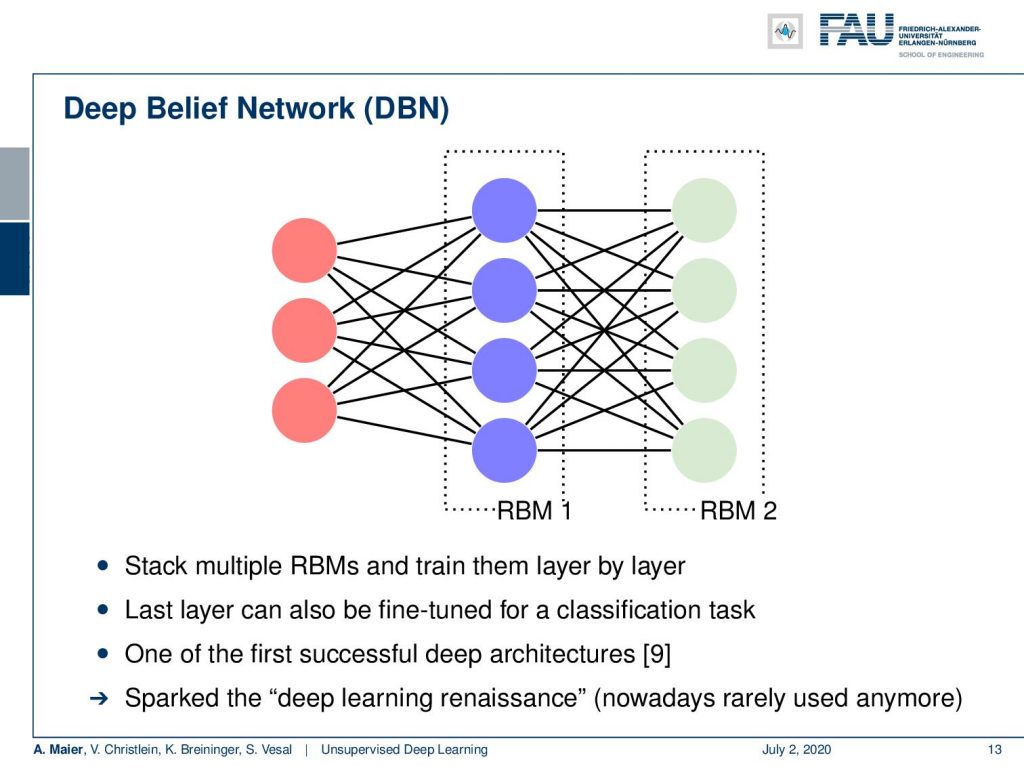RBMs on RBMs create Deep Believe Networks. Image under CC BY 4.0 from the Deep Learning Lecture.

You can expand on this into a deep belief network. The idea here is then that you stack layers on top again. The idea of deep learning is like layers on layers. So we need to go deeper and here we have one restricted Boltzmann machine on top another restricted Boltzmann machine. So, you can then use this to create really deep networks. One additional trick that you can use is that you use, for example, the last layer to fine-tune it for a classification task.

This is one of the first successful deep architectures as you see in . This sparked the deep learning renaissance. Nowadays, RMBs are rarely used. So, deep belief networks are not that commonly used anymore.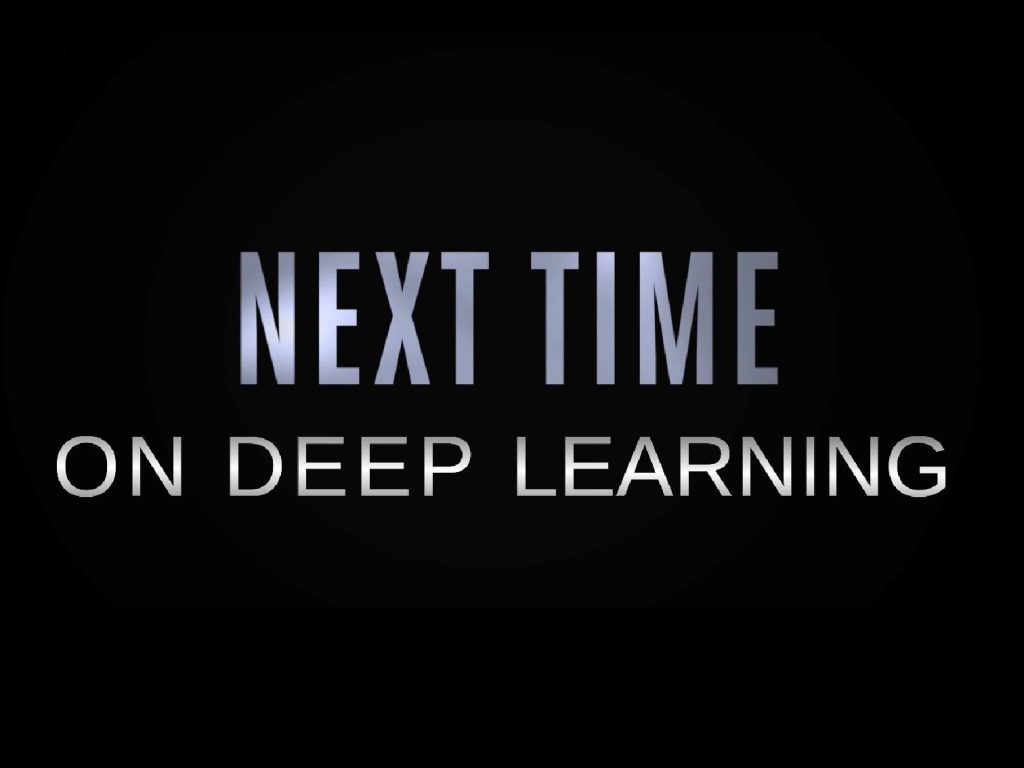More exciting things coming up in this deep learning lecture. Image under CC BY 4.0 from the Deep Learning Lecture.

So, this is the reason why we talk next time about autoencoders. We will look then in the next couple of videos into more sophisticated methods, for example, at the generative adversarial networks. So, I hope you liked this video and if you liked it then I hope to see you in the next one. Goodbye!

Link – NIPS 2016 GAN Tutorial of Goodfellow
Link – How to train a GAN? Tips and tricks to make GANs work (careful, not
everything is true anymore!)

## References

 Xi Chen, Xi Chen, Yan Duan, et al. “InfoGAN: Interpretable Representation Learning by Information Maximizing Generative Adversarial Nets”. In: Advances in Neural Information Processing Systems 29. Curran Associates, Inc., 2016, pp. 2172–2180.
 Pascal Vincent, Hugo Larochelle, Isabelle Lajoie, et al. “Stacked denoising autoencoders: Learning useful representations in a deep network with a local denoising criterion”. In: Journal of Machine Learning Research 11.Dec (2010), pp. 3371–3408.
 Emily L. Denton, Soumith Chintala, Arthur Szlam, et al. “Deep Generative Image Models using a Laplacian Pyramid of Adversarial Networks”. In: CoRR abs/1506.05751 (2015). arXiv: 1506.05751.
 Richard O. Duda, Peter E. Hart, and David G. Stork. Pattern classification. 2nd ed. New York: Wiley-Interscience, Nov. 2000.
 Asja Fischer and Christian Igel. “Training restricted Boltzmann machines: An introduction”. In: Pattern Recognition 47.1 (2014), pp. 25–39.
 John Gauthier. Conditional generative adversarial networks for face generation. Mar. 17, 2015. URL: http://www.foldl.me/2015/conditional-gans-face-generation/ (visited on 01/22/2018).
 Ian Goodfellow. NIPS 2016 Tutorial: Generative Adversarial Networks. 2016. eprint: arXiv:1701.00160.
 Martin Heusel, Hubert Ramsauer, Thomas Unterthiner, et al. “GANs Trained by a Two Time-Scale Update Rule Converge to a Local Nash Equilibrium”. In: Advances in Neural Information Processing Systems 30. Curran Associates, Inc., 2017, pp. 6626–6637.
 Geoffrey E Hinton and Ruslan R Salakhutdinov. “Reducing the dimensionality of data with neural networks.” In: Science 313.5786 (July 2006), pp. 504–507. arXiv: 20.
 Geoffrey E. Hinton. “A Practical Guide to Training Restricted Boltzmann Machines”. In: Neural Networks: Tricks of the Trade: Second Edition. Berlin, Heidelberg: Springer Berlin Heidelberg, 2012, pp. 599–619.
 Phillip Isola, Jun-Yan Zhu, Tinghui Zhou, et al. “Image-to-Image Translation with Conditional Adversarial Networks”. In: (2016). eprint: arXiv:1611.07004.
 Diederik P Kingma and Max Welling. “Auto-Encoding Variational Bayes”. In: arXiv e-prints, arXiv:1312.6114 (Dec. 2013), arXiv:1312.6114. arXiv: 1312.6114 [stat.ML].
 Jonathan Masci, Ueli Meier, Dan Ciresan, et al. “Stacked Convolutional Auto-Encoders for Hierarchical Feature Extraction”. In: Artificial Neural Networks and Machine Learning – ICANN 2011. Berlin, Heidelberg: Springer Berlin Heidelberg, 2011, pp. 52–59.
 Luke Metz, Ben Poole, David Pfau, et al. “Unrolled Generative Adversarial Networks”. In: International Conference on Learning Representations. Apr. 2017. eprint: arXiv:1611.02163.
 Mehdi Mirza and Simon Osindero. “Conditional Generative Adversarial Nets”. In: CoRR abs/1411.1784 (2014). arXiv: 1411.1784.
 Alec Radford, Luke Metz, and Soumith Chintala. Unsupervised Representation Learning with Deep Convolutional Generative Adversarial 2015. eprint: arXiv:1511.06434.
 Tim Salimans, Ian Goodfellow, Wojciech Zaremba, et al. “Improved Techniques for Training GANs”. In: Advances in Neural Information Processing Systems 29. Curran Associates, Inc., 2016, pp. 2234–2242.
 Andrew Ng. “CS294A Lecture notes”. In: 2011.
 Han Zhang, Tao Xu, Hongsheng Li, et al. “StackGAN: Text to Photo-realistic Image Synthesis with Stacked Generative Adversarial Networks”. In: CoRR abs/1612.03242 (2016). arXiv: 1612.03242.
 Han Zhang, Tao Xu, Hongsheng Li, et al. “Stackgan: Text to photo-realistic image synthesis with stacked generative adversarial networks”. In: arXiv preprint arXiv:1612.03242 (2016).
 Bolei Zhou, Aditya Khosla, Agata Lapedriza, et al. “Learning Deep Features for Discriminative Localization”. In: 2016 IEEE Conference on Computer Vision and Pattern Recognition (CVPR). Las Vegas, June 2016, pp. 2921–2929. arXiv: 1512.04150.
 Jun-Yan Zhu, Taesung Park, Phillip Isola, et al. “Unpaired Image-to-Image Translation using Cycle-Consistent Adversarial Networks”. In: CoRR abs/1703.10593 (2017). arXiv: 1703.10593.